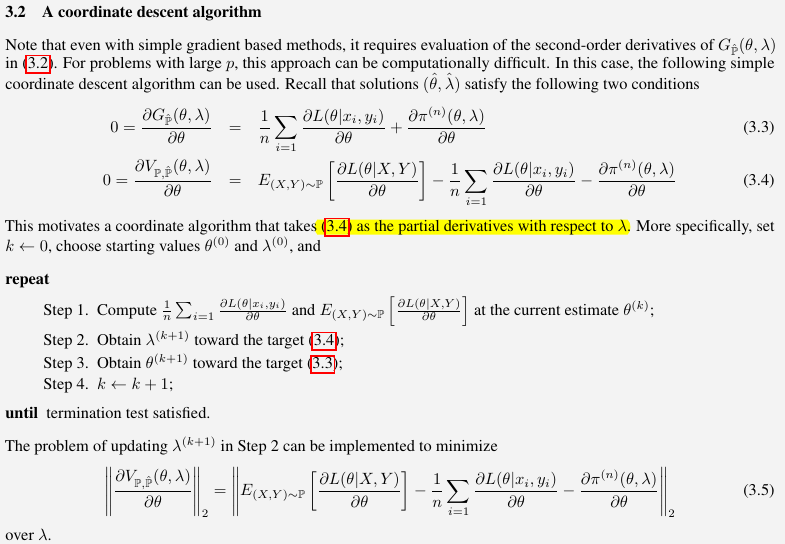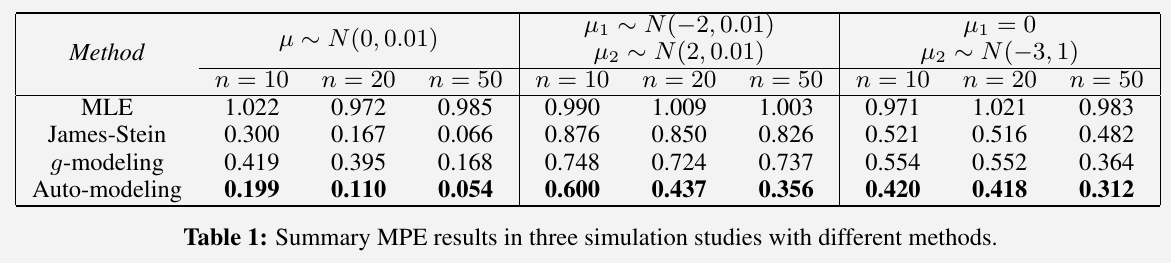# Fitting to Future Observations

##### Posted on Jul 21, 2022

sample $S={(x_i, y_i):i=1,\ldots,n}$ of measurements $(x, y)$ on each of $n$ items from a population $\bbP$ is modeled by

$Y\mid x \sim \bbP_{x, \theta}^{(n)}, Y\in \bbY, x\in \IR^q$

with unknown parameter $\theta\in \IR^p$.

Also write

$(X, Y)\sim \bbP_\theta^{(n)}, Y\in \cY, X\in \IR^q, \theta\in \IR^p$

where the distribution of $X$ is assumed to be independent of $\theta$

• $\ell(\theta\mid x, y)$: log-likelihood function of $\theta$, based on the model $\bbP_\theta^{(n)}$
• loss function: $L(\theta\mid x, y) = -\ell(\theta\mid x, y)$

Define the best model as $\bbP_{x,\theta}^{(n)}$ that minimizes the expected loss with respect to the population, that is, $\bbP_{x,\theta}$ with some $\theta$ in

$\Theta_\star = \left\{\theta: E_{(X, Y)\sim \bbP}[L(\theta\mid X, Y)]=\min_{\tilde\theta} E_{(X, Y)\sim \bbP}[L(\tilde \theta\mid X, Y)]\right\}$

A modelling strategy is said to be valid iff $$\lim_{n\rightarrow\infty}\bbP_\theta^{(n)}=\bbP$$ weakly for any $\theta\in \Theta_\star^{(n)}$ and some distance measure for probability distributions $\delta(\cdot,\cdot)$.

Consider two models $\bbP_{1,\theta}^{(n)}, \theta\in \Theta\subset \IR^{p_1}$, and $\bbP_{2,\xi}^{(n)}, \xi\in \Xi\subset \IR^{p_2}$, with bounded likelihood functions for a fixed sample size $n$ and some distance measure $\delta(\cdot,\cdot)$. Model 1 is said to be more efficient than Model 2 iff $E_{(X, Y)}\delta(\bbP_{1,\theta}^{(n)},\bbP)\le E_{X,Y}\delta(\bbP_{2,\xi}^{(n)},\bbP)$ for any $\theta \in \Theta_\star=\left\{\theta:E_{(X,Y)\sim \bbP}[L_1(\theta\mid X, Y)]=\min_{\tilde \theta}E_{(X,Y)\sim \bbP}[L_1(\tilde \theta\mid X, Y)]\right\}$ and $\xi \in \Xi_\star=\left\{\xi:E_{(X,Y)\sim \bbP}[L_2(\xi\mid X, Y)]=\min_{\tilde\xi}E_{(X,Y)\sim \bbP}[L_2(\tilde \xi\mid X, Y)]\right\}$, where $L_1$ and $L_2$ are the loss functions of model $\bbP_{1,\theta}^{(n)}$ and $\bbP_{2,\xi}^{(n)}$, respectively.

Different from Box’s “All models are wrong but some are useful”, the paper said “models, as simple-but-not-simpler approximations to $\bbP$, depend on the observed data and often change as more data are available.”

The definition of efficiency emphasises on the importance of modeling for future observations and suggests in auto-modeling with over-parameterized models, an imputation method be used to approximate the population.

## An Imputation and Estimation Algorithm

Find an approximate solution to the desired optimization problem

$\hat\theta = \arg\min_\theta E_{(X, Y)\sim \bbP} [L(\theta\mid X, Y)]$

by optimizing its sample counterpart, with a preference for simple models to control the deviation of the estimate of $\theta$,

$G_{\hat \bbP}(\theta, \lambda) = E_{(X, Y)\sim \hat \bbP}[L(\theta\mid X, Y)]+\pi^{(n)}(\theta,\lambda) = \frac 1n \sum_{i=1}^n L(\theta\mid x_i, y_i) + \pi^{(n)}(\theta,\lambda)\,,$

where $\hat\bbP$ is the empirical distribution defined by the sample ${(x_i, y_i):i=1,\ldots,n}$.

Take a type of generalized $L_1$ penalty,

$\pi_\lambda^{(n)}(\theta)=\sum_i\lambda_i\vert \theta_i\vert\,, \lambda_i\ge 0, i=1,\ldots,n\,.$

Write the target objective function,

$E_{(X, Y)\sim \bbP}[L(\theta\mid X, Y)] = G_{\hat\bbP}(\theta,\lambda) + V_{\bbP,\hat \bbP}(\theta,\lambda)$

where

$V_{\bbP,\hat\bbP}(\theta,\lambda) = E_{(X,Y)\sim \bbP}[L(\theta\mid X, Y)] - E_{(X, Y)\sim \hat\bbP}[L(\theta\mid X, Y)] - \pi^{(n)}(\theta,\lambda)\,.$

$G_{\hat \bbP}(\theta, \lambda)$ is minimized over $\theta$ at $\theta = \hat\theta$ for given $\lambda$, the remaining term $V_{\bbP,\hat\bbP}(\theta,\lambda)$ serves as a measure of validation.

Desire to choose $\lambda$ to minimize $E_{(X, Y)\sim \bbP}[L(\theta\mid X, Y)]$, then looks for a solution to have

$\frac{\partial V_{\bbP,\hat \bbP}(\theta,\lambda)}{\partial \theta_i}=0\,.$

for all $i=1,\ldots, p$.

Consider a weaker alternative condition: $V_{\bbP,\hat\bbP}(\theta,\lambda)$ is required to be approximately a constant in a neighborhood of $\hat \theta$. (why?)

$\hat \lambda_i = \min_{\lambda_i} \left\vert \frac{\partial V_{\bbP,\hat\bbP}(\theta,\lambda)}{\partial \theta_i} \right\vert$

for all $i=1,\ldots,p$.

is $\hat \lambda_i$ a function of $\theta_i$?

Create one future observation $(x, y)$

1. take a bootstrap sample ${(\tilde x_i, \tilde y_i):i=1,\ldots, n}$. Denote by $\tilde \bbP$ the corresponding empirical distribution.
2. find the bootstrap estimate $\tilde\theta$, that is, $\tilde\theta$ is obtained by minimizing $G_{\hat \bbP}(\theta,\lambda)$ over $\theta$ subject to
$\hat \lambda_i = \min_{\lambda_i} \left\vert \frac{\partial V_{\hat\bbP,\tilde\bbP}(\theta,\lambda)}{\partial \theta_i} \right\vert, i=1,\ldots,p$
1. draw $x$ from ${x_i:i=1,\ldots,n}$ at random and simulate $y$ from the fitted predictive model

## Numerical Optimization Methods

Find a pair of solutions $\hat\theta$ and $\hat\lambda$ that minimize both $G_{\hat \bbP}(\theta,\lambda)$ and $V_{\bbP,\hat\bbP}(\theta,\lambda)$, as functions of $\theta$ (???), at $\theta=\hat\theta$ for some $\lambda=\hat\lambda$.

### Method of Lagrange multipliers

$\min_{\theta, \lambda} V_{\bbP,\hat\bbP}(\theta,\lambda)$

subject to the system of constraints $\partial G_{\hat\bbP}(\theta,\lambda)/\partial\theta=0$. The Lagrange function of $(\theta,\lambda,\phi)$ to be minimized becomes

$g(\theta, \lambda,\phi) = V_{\bbP,\hat \bbP}(\theta,\lambda) + \phi'\frac{\partial G_{\hat \bbP}(\theta,\lambda)}{\partial \theta}$

### Coordinate Descent Algorithm• Take another perspective of (3.3), it is just to minimize $G_{\hat\bbP}(\theta, \lambda)$. Thus, we can directly take advantage with existing nonlinear programming solvers, such as Ipopt (Interior Point Optimizer).
• For (3.4) and (3.5), since $\theta_2,\cdots,\theta_m\ge 0$, then
$\pi^{(n)}(\theta,\lambda) = \sum_{k=2}^m \lambda_k\vert \theta_k\vert = \sum_{k=2}^m \lambda_k\theta_k$

and hence

$\frac{\partial \pi^{(n)}(\theta,\lambda)}{\partial \theta} = % \begin{bmatrix} % 0 \\ % \lambda_2\\ % \vdots\\ % \lambda_m\\ % 0\\ % \vdots\\ % 0 % \end{bmatrix} \begin{bmatrix} 0 & \lambda_2 & \lambda_3 & \cdots & \lambda_m & 0 & \cdots & 0 \end{bmatrix}^T$

## Application: Many-Normal-Means

Make inference on the unknown means $\mu_1,\ldots, \mu_n$ from the sample $Y_1,\ldots, Y_n$ with the model $Y_i\mid {\mu_1,\ldots,\mu_n}\sim N(\mu_i,1),i=1,\ldots,n$, where $Y_1,\ldots,Y_n$ are independent of each other.

Re-formulate the problem: $Y_1,\ldots,Y_n$ is assumed to be a sample from a single population and view $\mu_1,\ldots,\mu_n$ as missing values taken from some unknown population. That is,

$\mu_i\sim \bbP_\theta^{(n)}\qquad\text{and}\qquad Y_i\mid\mu_i\sim N(\mu_i,1)$

Take a simple discrete distribution at $m\ge n$ unknown points $\eta_1\le \eta_2\le\cdots\le \eta_m$ with the probability mass $\Pr(\mu = \eta_k) = \alpha_k, k=1,\ldots,m$. Then the observed-data likelihood based on $Y_i$ is given by $1/{\sqrt{2\pi}}\sum_{k=1}^m\alpha_k\exp(-\frac{(Y_i-\eta_k)^2}{2})$

Auto-modeling framework is used for fitting the discrete distribution model $\bbP_\theta^{(n)}$, which provides an approximation to the true distribution.

For simplicity-preference function, write $\theta_1=\eta_1, \theta_k=\eta_k-\eta_{k-1}$ for $k=2,\ldots,m$ and $\theta_{m+k}=\alpha_k$ for $k=1,\ldots,m$. Take the $L_1$ penalty on $(\theta_2,\ldots,\theta_m)’\in \IR^{m-1}$, that is $\pi_\lambda^{(n)}(\theta) = \sum_{k=2}^m\lambda_k\vert \theta_k\vert$, where $\lambda_k\ge 0$ and $k=2,\ldots, m$.

\begin{align*} L_i \triangleq L(\theta\mid y_i) &= -\log \sum_{k=1}^m \alpha_k \exp\left(-\frac{(y_i-\eta_k)^2}{2}\right)\\ &= -\log \sum_{k=1}^m \theta_{m+k} \exp\left(-\frac{(y_i-\sum_{j=1}^k\theta_j)^2}{2}\right)\,, \end{align*}

then

$\frac{\partial L_i}{\partial \theta_\ell} = \begin{cases} -\frac{\sum_{k=\ell}^m\theta_{m+k}\exp\left(-\frac{(y_i-\sum_1^k\theta_j)^2}{2}\right)(y_i-\sum_1^k\theta_j)}{\sum_{k=1}^m\theta_{m+k}\exp\left(-\frac{(y_i-\sum_1^k\theta_j)^2}{2}\right)} & \ell\le m\\ -\frac{\exp\left(-\frac{(y_i-\sum_1^{\ell-m}\theta_j)^2}{2}\right)}{\sum_{k=1}^{m}\theta_{m+k}\exp\left(-\frac{(y_i-\sum_1^k\theta_j)^2}{2}\right)}& \ell\ge m+1 \end{cases}$

Alternatively, we can use finite difference to approximate the derivatives, such as the JuliaDiff/FiniteDifferences.jl package in Julia, but attention that forward_fdm might be needed due to singularity issue of log function.

• MLE: $\hat\mu^{MLE}_i=y_i$.

• James-Stein Estimator:

$\hat\mu^{JS}_i = \bar y + \left[1-\frac{n-3}{\sum(y_i-\bar y)^2}\right](y_i-\bar y)\,,$

which shrinks the MLE of each $\mu$ towards the overall sample mean of the observed data.

• g-modeling: According to Narasimhan and Efron (2020), Empirical Bayes inference assumes an unknown prior density $g(\theta)$ has yield (unobservable) $\Theta_1,\ldots,\Theta_N$, and each $\Theta_i$ produces an independent observation $X_i$ from $p_i(X_i\mid \Theta_i)$. The marginal density $f_i(X_i)$ is a convolution of the prior $g$ and $p_i$. The Bayes deconvolution problem is to recover $g$ from the data. Generally, the estimation of $g$, so-called g-modeling, is difficult, but Narasimhan and Efron (2020) proposed to restrict the prior $g$ to lie within a parametric family of distributions and claimed that the results are more encouraging. Their R package deconvolveR is available on CRAN.

For the many normal means example, just call

result <- deconv(tau = tau, X = x, deltaAt = 0, family = "Normal")


where x is the observed data, and tau is a vector of discrete support points for $\theta$.For simplicity, I skip the step of approximation of $\bbP$ by bootstrap samples, and instead directly use the true $\bbP$. My results are as follows,

julia> @time many_normal_means(n=10,K=200,case=1)
409.362780 seconds (3.79 G allocations: 408.156 GiB, 13.74% gc time)
1×4 Matrix{Float64}:
0.951092  0.297215  0.18758  0.174413

julia> @time many_normal_means(n=10,K=200,case=2)
1166.330146 seconds (10.30 G allocations: 1.085 TiB, 14.45% gc time)
1×4 Matrix{Float64}:
1.05033  0.948022  0.849796  0.684122

julia> @time many_normal_means(n=10,K=200,case=3)
873.212648 seconds (7.60 G allocations: 818.290 GiB, 14.50% gc time, 1.37% compilation time)
1×4 Matrix{Float64}:
1.01645  0.565332  0.428163  0.438326

julia> @time many_normal_means(n=20,K=200,case=1)
1506.267866 seconds (11.68 G allocations: 1.780 TiB, 14.42% gc time)
1×4 Matrix{Float64}:
1.0176  0.151387  0.0856947  0.107758

julia> @time many_normal_means(n=20,K=200,case=2)
5058.591486 seconds (38.67 G allocations: 5.893 TiB, 13.97% gc time, 0.00% compilation time)
1×4 Matrix{Float64}:
0.994893  0.85625  0.764716  0.488117

julia> @time many_normal_means(n=20,K=200, case=3)
4469.796364 seconds (32.48 G allocations: 4.950 TiB, 14.42% gc time)
1×4 Matrix{Float64}:
1.05389  0.511735  0.323881  0.460926


We observe that

• Except the results of g-modeling, others are close to the reported results in the paper.
• Sometimes g-modeling can outperform auto-modeling, but I did not deliberately select its parameters. The paper also did not discuss how they choose the parameters for this method.
• Currently the program is quite slow, and the computational burden is mainly Step 3 in the coordinate descent algorithm. Is there any speed-up strategies?